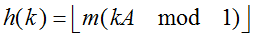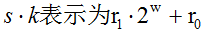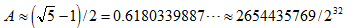﻿ 乘法散列法

乘法散列法k: 关键字
A: 常数 (0 < A < 1)
kA mod 1: 取kA的小数部分
m: 一般选择它为2的某个幂次(m=2p)h(k)=对r0取p个最高有效位(即，h(k)=r0>>(w-p))
A一般取下面的这个值比较理想```using System;
using System.Collections.Generic;
using System.Linq;
using System.Text;
/// <summary>
/// 乘法散列法 测试
/// </summary>
namespace HashTest
{
class Program
{
static void Main(string[] args)
{
for(int i=123454; i< 123459; i++)
{
Console.WriteLine("hash1 ({0})={1}", i, CalHash1(i));
Console.WriteLine("hash2 ({0})={1}", i, CalHash2(i));
}

//注: 由于方案一中的A值与方案二中选的s值存在精度差，所以部分数据计算的结果不相同。

}

//计算方案一
public static double CalHash1(int k)
{
int p = 14;
//选一个0-1之间的小数
double A = 0.6180339887;//(Math.Sqrt(5) - 1) / 2;
//选一个2的幂次方的m值
double m = Math.Pow(2, p);
//直接套用公式计算
double hash = Math.Floor(m * (k * A % 1));
return hash;
}

//计算方案二 (优化版) 推荐
public static int CalHash2(int k)
{
double s = 2654435769;//推荐值
double ks = k * s;
int r0 = (int)(ks % int.MaxValue);//余数
int hash = r0 >> 18;//取高14位 (可以根据实际的存储大小调整取高几位)
return hash;
}
}
}
```